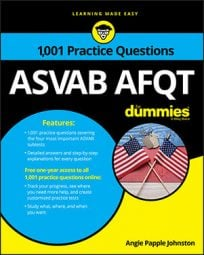##### ASVAB AFQTJust as there are different sides to every story, there are also different sides to every three-dimensional object. Some questions on the Mathematics Knowledge subtest on the ASVAB will ask you to add up all the sides of a figure to find its total surface area.

## Practice questions

1. Find the surface area:

A. 48 square yards B. 72 square yards C. 90 square yards D. 96 square yards

2. Find the surface area:

A. 2,800 cm2 B. 2,820 cm2 C. 2,826 cm2 D. 2,152 cm2

1. The correct answer is Choice (D).

The formula to find the surface area of a prism is SA = 2B + Ph, where B represents the area of the base, P represents the perimeter of the base, and h represents the height of the prism.

First find the area of the triangular base using

where b represents the triangle's base and h represents its height:

Find its perimeter by adding the length of each leg:

6 + 8 + 10 = 24

The base's area is 24 square yards, the base's perimeter is 24 yards, and the prism's height is 2 yards, so plug that information into the formula for the surface area of a prism:

SA = 2(24) + 24(2)

SA = 48 + 48

SA = 96

The surface area of the prism is 96 square yards.

2. The correct answer is Choice (C).

The formula to find the surface area of a sphere is

so use the values you know to solve the problem: